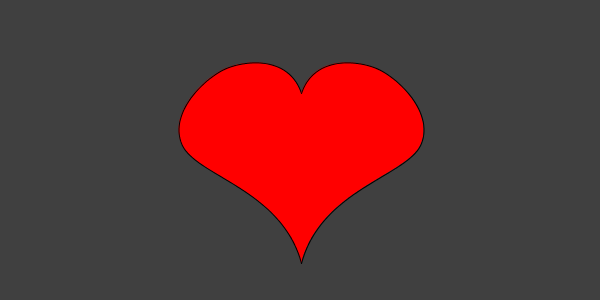# Scaling Heart

example processingThis program uses the `width` and `height` variables along with the `curveVertex()` function to draw a heart:

``````size(300, 300);

background(64);

fill(255, 0, 0);

//left half of heart
beginShape();
curveVertex(width/2, height*2);
curveVertex(width/2, height*.9);
curveVertex(width*.1, height/2);
curveVertex(width/4, height/4);
curveVertex(width/2, height/3);
curveVertex(width/2, height);
endShape();

//right half of heart
beginShape();
curveVertex(width/2, height);
curveVertex(width/2, height/3);
curveVertex(width*.75, height/4);
curveVertex(width*.9, height/2);
curveVertex(width/2, height*.9);
curveVertex(width/2, height*2);
endShape();
``````Code Editor

See the Pen by Happy Coding (@KevinWorkman) on CodePen.

The `curveVertex()` is a little weird, because it’s not exactly a “direct” drawing function like the `ellipse()` or `rect()` functions. With the `ellipse()` and `rect()` functions, you pass in parameters and Processing draws a shape at those coordinates. But with the `curveVertex()` function, it’s more like you’re steering the curves than directly drawing them. The first call to `curveVertex()` sets up an anchor point, but the curve doesn’t actually start drawing until the second call to `curveVertex()`. It will curve around the points you pass in, and the last call to `curveVertex()` is another guiding point that determines which direction the line should curve.

If none of that makes sense, that’s okay. It takes me a long time to understand curves too (you should have seen all the misshapen hearts I drew trying to figure this example out). As always, try passing in different parameters to see what happens!

## Tweak Ideas

• Change the color of the heart. Make it a random color!
• Add somebody’s name to the middle of the heart. Send them a nerdy Valentine!